# 51. N-Queens

Reference: LeetCode
Difficulty: Hard

My Post:

## Problem

The n-queens puzzle is the problem of placing n queens on an n×n chessboard such that no two queens attack each other.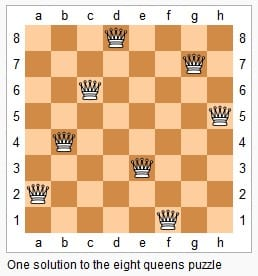Given an integer n, return all distinct solutions to the n-queens puzzle.

Each solution contains a distinct board configuration of the n-queens’ placement, where Q and . both indicate a queen and an empty space respectively.

Note: A queen can attack other queens that are at the same row or column or at the diagonal line.

Example:

## Analysis

### Brute-Force

Enumerate all possible placements of queens on a nxn chessboard and check if each is valid.

Time: $O(N^N)$
Space: $O(1)$ if we do not consider the output list.

### Backtracking

The basic idea is to examine each row and use an array attack to restrict the possibilities in the future searching.

• Why will we stop? In other words, what is the base case?
• How do we make string generation more efficiently? StringBuilder
• Why should we initialize attack array with int type rather than boolean?

The graph is from LeetCode solution section.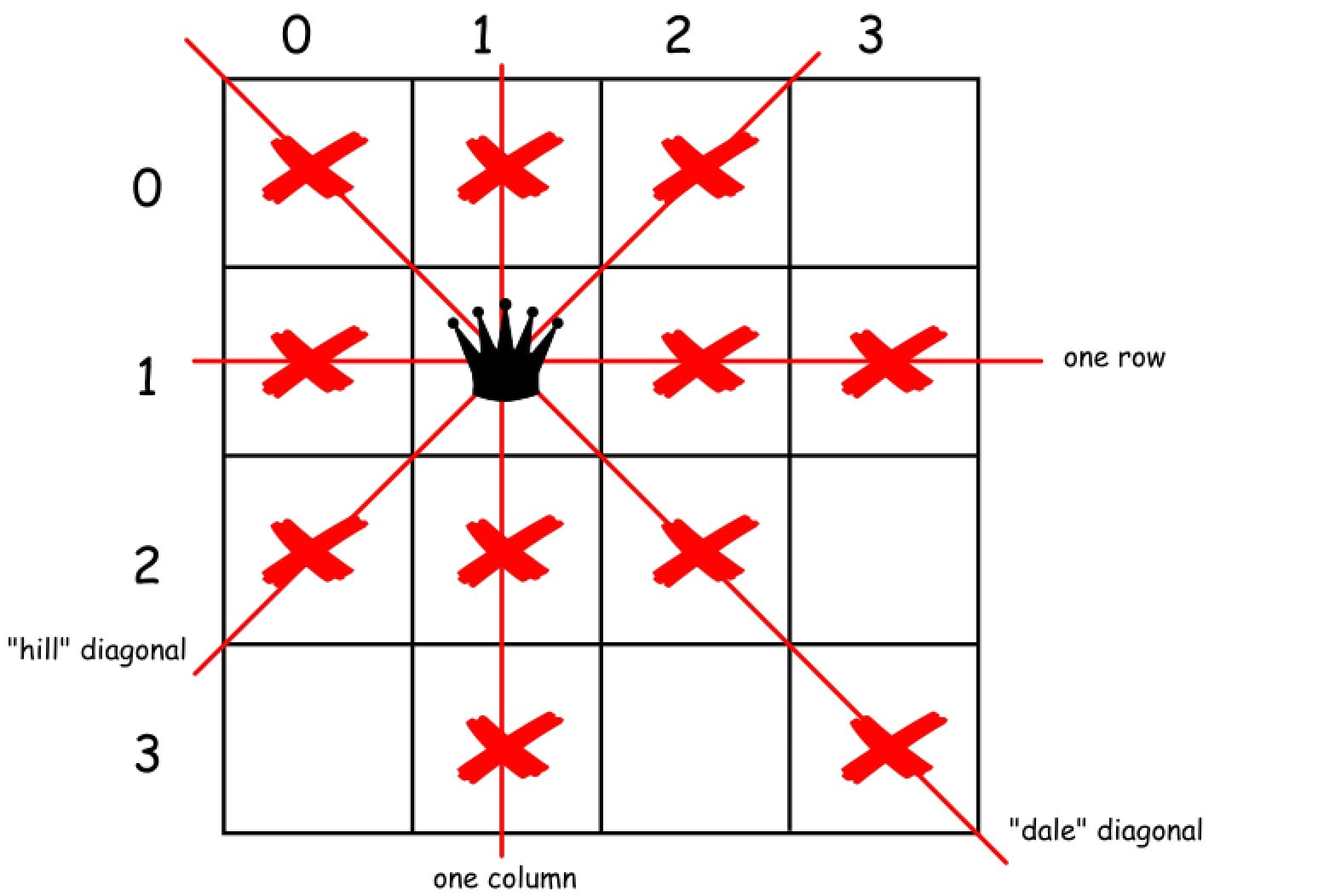Each time we pick a queen (i, j) we need to update all the attacked positions below it, which include three cases as shown in the graph:

• Left-Below positions
• Below positions
• Right-Below positions

Notice that we cannot use boolean since some attacked positions might be overlapped.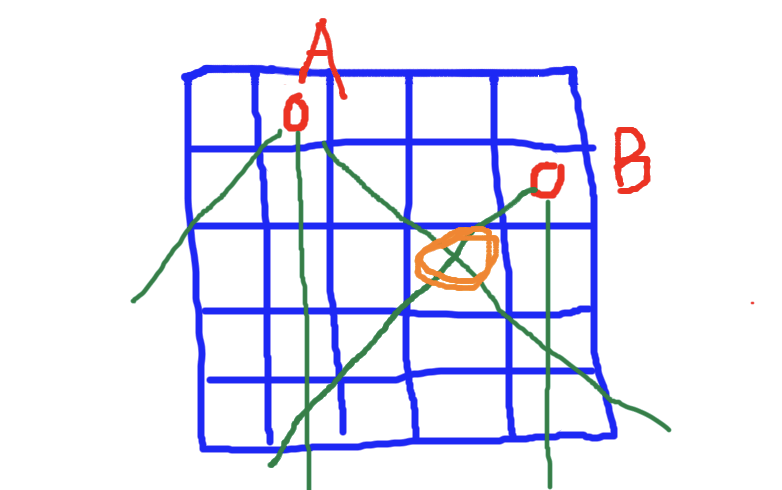In the example above, when we restore attacked position for queen B, the orange position will be restored to no-attack state if we use true/false; however, it is still under attack by queen A.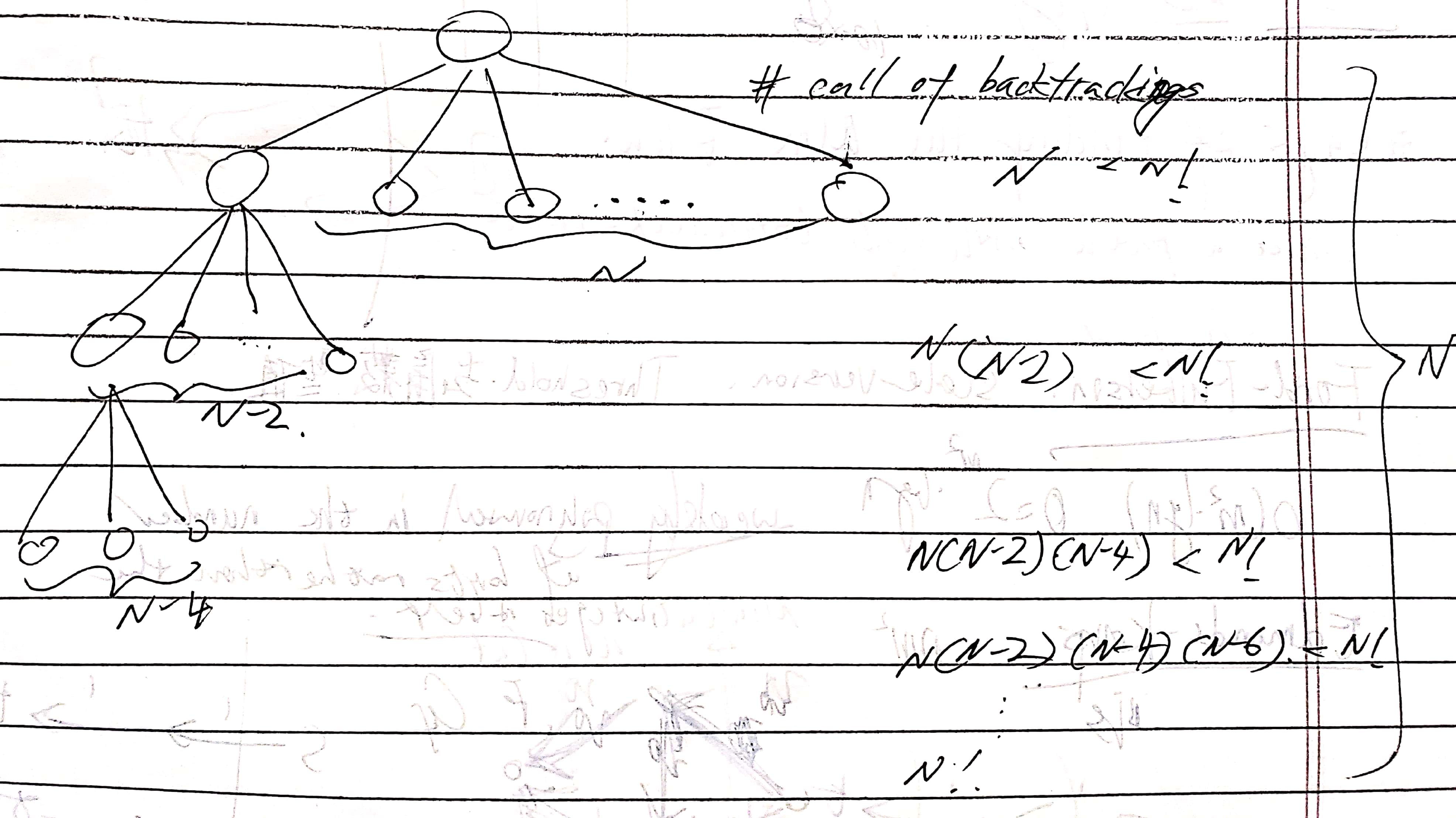Time: $O(N \times N!)$. There is $N$ possibilities to put the first queen, then no more than $N(N-2)$ to put the second one, and no more than $N(N-2)(N-4)$ to put the third one, and so forth. In total, there are $N$ layers. The number of calls of backtracking at each layer is upper bounded by $N!$. (not consider string construction)
Space: $O(N^2)$ since there are call stacks and attack array (do not consider output).

The space complexity can be optimized by using $N$-size array rows, dale, and hill. Each element of them denotes a specific vertical line or diagonal line that a queen can attack.

From LeetCode solution section: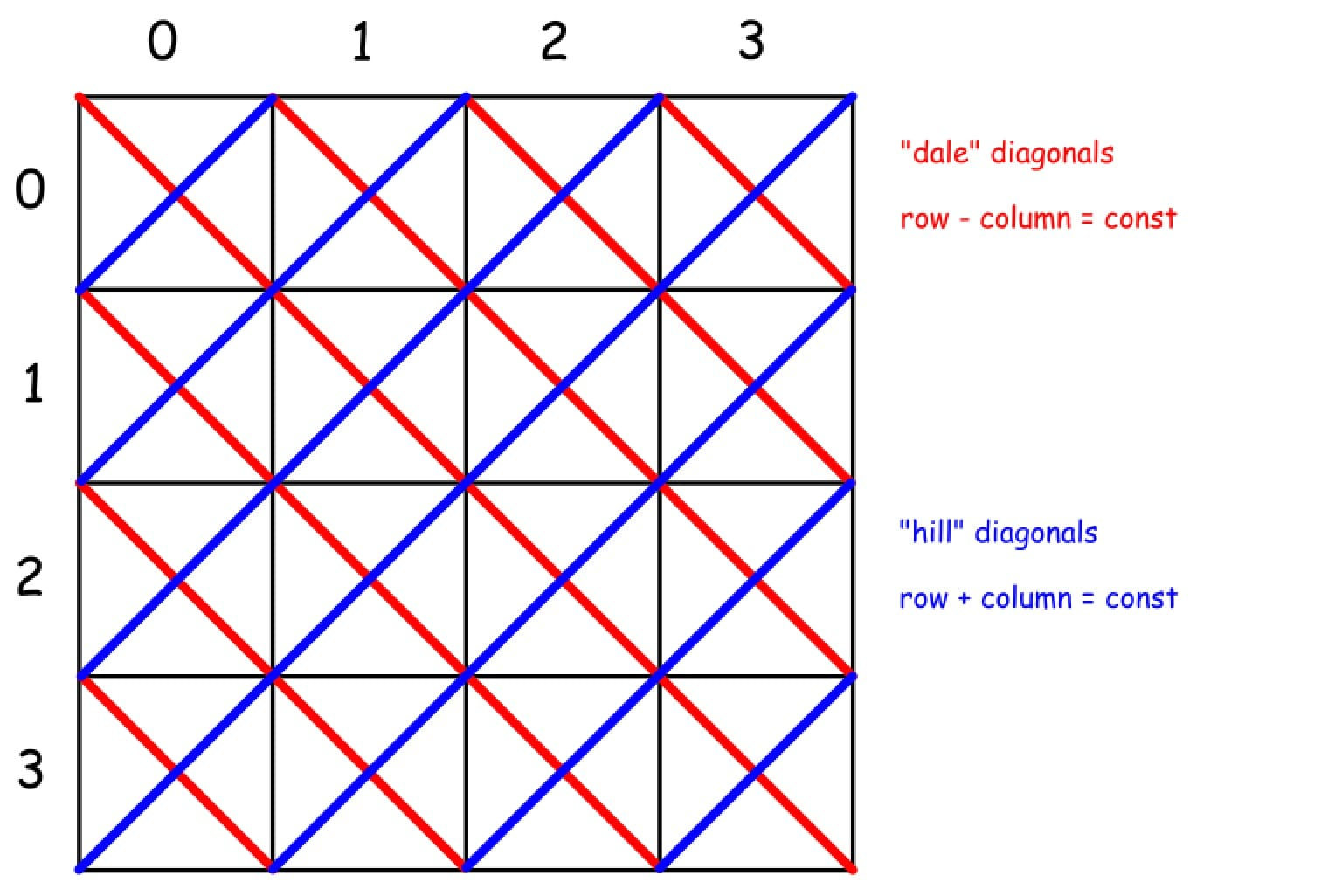## N-Queens II

Returns the number of solutions. Modify based on the above solution.

CommentJunhao Wang
a software engineering cat